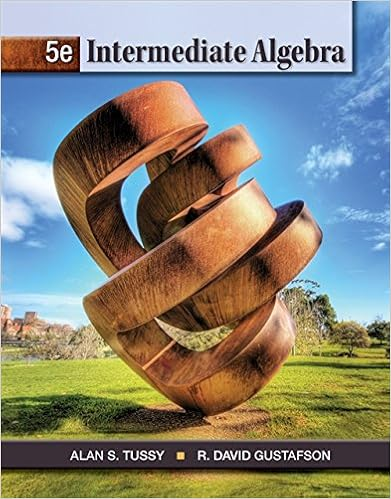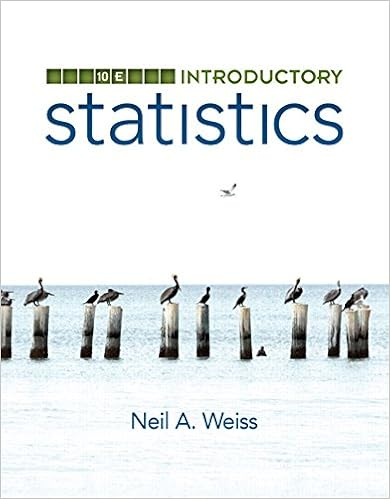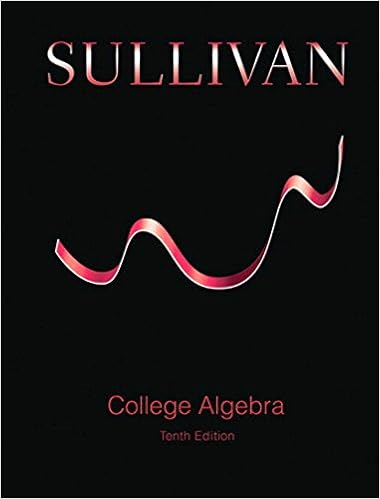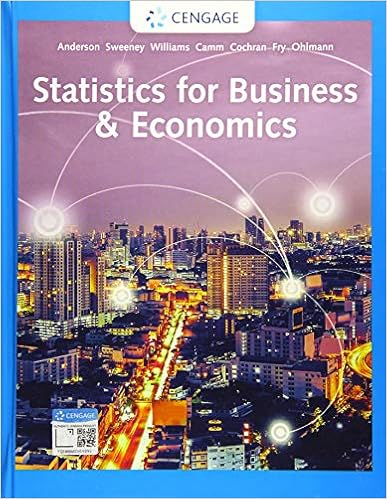# Differential Equations Exam Review (2 of 6) - Review 1:...

• Test Prep
• 3

This preview shows page 1 - 2 out of 3 pages.

Review 1: L1-L71.Determine which of the equations given below (the independent variable is specified)is1) linear;2) separable;3) both linear and separable;4) neither linear nor separable.A.3tdxxt xedt+=B.211dyxyxdx=+C.0ydyxdx=D.xdyxedx=(t – independent)(x – independent)(x – independent)(x – independent)2. Verify whether the function()21xxxφ=+is an explicit solution to the differentialequation2222d yydxx=.3.Determine whether the given relation is an implicit solution to the differentialequation:32lnxyy=232lndyx ydxyy=+4. Determine whether the Uniqueness and Existence Theorem implies that the giveninitial value problem has a unique solution.45.Solve the initial value problem in #4. How many solutions do you have? Give each
solution in explicit form.6. Determine whether the Uniqueness and Existence Theorem for a linear first-orderdifferential equation implies that the given initial value problem has a unique solution. If

Course Hero member to access this document

##### We have textbook solutions for you!The document you are viewing contains questions related to this textbook.
Chapter 6 / Exercise 10
Intermediate Algebra
Tussy/GustafsonExpert VerifiedBrowse all Textbook Solutions

Course Hero member to access this document

End of preview. Want to read all 3 pages?

Course Hero member to access this document

Term
Fall
Professor
TUNCER
##### We have textbook solutions for you!
The document you are viewing contains questions related to this textbook.The document you are viewing contains questions related to this textbook.
Chapter 6 / Exercise 10
Intermediate Algebra
Tussy/GustafsonExpert Verified
•••